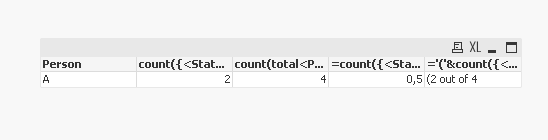# New to QlikView

Discussion board where members can get started with QlikView.

Announcements
Modernize Your QlikView Deployment webinar, Nov. 3rd. REGISTER
cancel
Showing results for
Did you mean:
HighlightedContributor

## Text Based Measure

Is it possible to place a text based measure into a table field without having to calculate it at the load stage (and if that was the only way what sort of syntax would i need to create it).

For example, i have a number of rows of training data

Person, Course, Status

A, Course 1, Complete
A, Course 2, Complete
A, Course 3, Pending
A, Course 4, Rejected

Column A on my table is the person, in this example A, i would like to them count the rows for that person that are complete, and the total rows, and then in a column display the number of complete vs total, i.e. "2/4" in this example. (2 out of 4)

1 Solution

Accepted Solutions
HighlightedMaster

@DMG if I understood correctly

Dimension : Pearson

Measure, you can use for example :

count({<Status={'Complete'}>} Course) for count the rows for that person that are complete

count(total<Person> Course) for total rows for that pearson

and =count({<Status={'Complete'}>} Course)/count(total<Person> Course) the number of complete vs total

if you want you can use the text format as :

='('&count({<Status={'Complete'}>} Course)&' out of '&count(total<Person> Course)

output:Regards,
Taoufiq ZARRA

"Please LIKE posts and "Accept as Solution" if the provided solution is helpful "

(you can mark up to 3 "solutions") 😉
2 Replies
HighlightedMaster

@DMG if I understood correctly

Dimension : Pearson

Measure, you can use for example :

count({<Status={'Complete'}>} Course) for count the rows for that person that are complete

count(total<Person> Course) for total rows for that pearson

and =count({<Status={'Complete'}>} Course)/count(total<Person> Course) the number of complete vs total

if you want you can use the text format as :

='('&count({<Status={'Complete'}>} Course)&' out of '&count(total<Person> Course)

output:Regards,
Taoufiq ZARRA

"Please LIKE posts and "Accept as Solution" if the provided solution is helpful "

(you can mark up to 3 "solutions") 😉
HighlightedMVP

@DMG  you can try creating table with dimension person and below expression

=Count({<Status={'Complete'}>}Status) '/'& Count(Status)